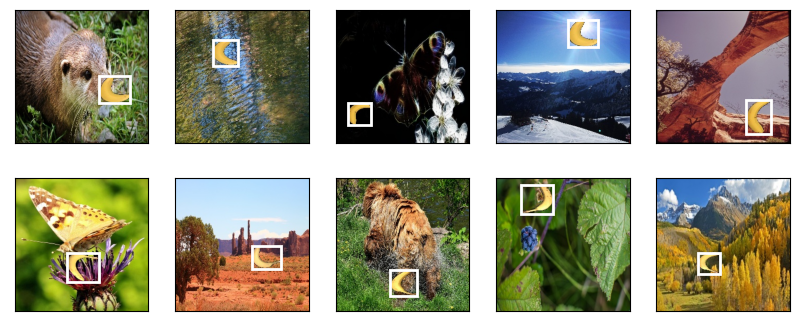# 13.6. The Object Detection Dataset¶ Open the notebook in Colab Open the notebook in Colab Open the notebook in Colab Open the notebook in SageMaker Studio Lab

There is no small dataset such as MNIST and Fashion-MNIST in the field of object detection. In order to quickly demonstrate object detection models, we collected and labeled a small dataset. First, we took photos of free bananas from our office and generated 1000 banana images with different rotations and sizes. Then we placed each banana image at a random position on some background image. In the end, we labeled bounding boxes for those bananas on the images.

The banana detection dataset with all the image and csv label files can be downloaded directly from the Internet.

%matplotlib inline
import os
import pandas as pd
from mxnet import gluon, image, np, npx
from d2l import mxnet as d2l

npx.set_np()

#@save
d2l.DATA_HUB['banana-detection'] = (
d2l.DATA_URL + 'banana-detection.zip',
'5de26c8fce5ccdea9f91267273464dc968d20d72')

%matplotlib inline
import os
import pandas as pd
import torch
import torchvision
from d2l import torch as d2l

#@save
d2l.DATA_HUB['banana-detection'] = (
d2l.DATA_URL + 'banana-detection.zip',
'5de26c8fce5ccdea9f91267273464dc968d20d72')


We are going to read the banana detection dataset in the read_data_bananas function below. The dataset includes a csv file for object class labels and ground-truth bounding box coordinates at the upper-left and lower-right corners.

#@save
"""Read the banana detection dataset images and labels."""
csv_fname = os.path.join(data_dir, 'bananas_train' if is_train
else 'bananas_val', 'label.csv')
csv_data = csv_data.set_index('img_name')
images, targets = [], []
for img_name, target in csv_data.iterrows():
os.path.join(data_dir, 'bananas_train' if is_train else
'bananas_val', 'images', f'{img_name}')))
# Here target contains (class, upper-left x, upper-left y,
# lower-right x, lower-right y), where all the images have the same
# banana class (index 0)
targets.append(list(target))
return images, np.expand_dims(np.array(targets), 1) / 256

#@save
"""Read the banana detection dataset images and labels."""
csv_fname = os.path.join(data_dir, 'bananas_train' if is_train
else 'bananas_val', 'label.csv')
csv_data = csv_data.set_index('img_name')
images, targets = [], []
for img_name, target in csv_data.iterrows():
os.path.join(data_dir, 'bananas_train' if is_train else
'bananas_val', 'images', f'{img_name}')))
# Here target contains (class, upper-left x, upper-left y,
# lower-right x, lower-right y), where all the images have the same
# banana class (index 0)
targets.append(list(target))
return images, torch.tensor(targets).unsqueeze(1) / 256


By using the read_data_bananas function to read images and labels, the following BananasDataset class will allow us to create a customized Dataset instance for loading the banana detection dataset.

#@save
class BananasDataset(gluon.data.Dataset):
"""A customized dataset to load the banana detection dataset."""
def __init__(self, is_train):
print('read ' + str(len(self.features)) + (f' training examples' if
is_train else f' validation examples'))

def __getitem__(self, idx):
return (self.features[idx].astype('float32').transpose(2, 0, 1),
self.labels[idx])

def __len__(self):
return len(self.features)

#@save
class BananasDataset(torch.utils.data.Dataset):
"""A customized dataset to load the banana detection dataset."""
def __init__(self, is_train):
print('read ' + str(len(self.features)) + (f' training examples' if
is_train else f' validation examples'))

def __getitem__(self, idx):
return (self.features[idx].float(), self.labels[idx])

def __len__(self):
return len(self.features)


Finally, we define the load_data_bananas function to return two data iterator instances for both the training and test sets. For the test dataset, there is no need to read it in random order.

#@save
batch_size, shuffle=True)
batch_size)
return train_iter, val_iter

#@save
batch_size, shuffle=True)
batch_size)
return train_iter, val_iter


Let us read a minibatch and print the shapes of both images and labels in this minibatch. The shape of the image minibatch, (batch size, number of channels, height, width), looks familiar: it is the same as in our earlier image classification tasks. The shape of the label minibatch is (batch size, $$m$$, 5), where $$m$$ is the largest possible number of bounding boxes that any image has in the dataset.

Although computation in minibatches is more efficient, it requires that all the image examples contain the same number of bounding boxes to form a minibatch via concatenation. In general, images may have a varying number of bounding boxes; thus, images with fewer than $$m$$ bounding boxes will be padded with illegal bounding boxes until $$m$$ is reached. Then the label of each bounding box is represented by an array of length 5. The first element in the array is the class of the object in the bounding box, where -1 indicates an illegal bounding box for padding. The remaining four elements of the array are the ($$x$$, $$y$$)-coordinate values of the upper-left corner and the lower-right corner of the bounding box (the range is between 0 and 1). For the banana dataset, since there is only one bounding box on each image, we have $$m=1$$.

batch_size, edge_size = 32, 256
batch = next(iter(train_iter))
batch.shape, batch.shape

Downloading ../data/banana-detection.zip from http://d2l-data.s3-accelerate.amazonaws.com/banana-detection.zip...

((32, 3, 256, 256), (32, 1, 5))

batch_size, edge_size = 32, 256
batch = next(iter(train_iter))
batch.shape, batch.shape

Downloading ../data/banana-detection.zip from http://d2l-data.s3-accelerate.amazonaws.com/banana-detection.zip...

(torch.Size([32, 3, 256, 256]), torch.Size([32, 1, 5]))


## 13.6.3. Demonstration¶

Let us demonstrate ten images with their labeled ground-truth bounding boxes. We can see that the rotations, sizes, and positions of bananas vary across all these images. Of course, this is just a simple artificial dataset. In practice, real-world datasets are usually much more complicated.

imgs = (batch[0:10].transpose(0, 2, 3, 1)) / 255
axes = d2l.show_images(imgs, 2, 5, scale=2)
for ax, label in zip(axes, batch[0:10]):
d2l.show_bboxes(ax, [label[1:5] * edge_size], colors=['w'])imgs = (batch[0:10].permute(0, 2, 3, 1)) / 255
axes = d2l.show_images(imgs, 2, 5, scale=2)
for ax, label in zip(axes, batch[0:10]):
d2l.show_bboxes(ax, [label[1:5] * edge_size], colors=['w'])## 13.6.4. Summary¶

• The banana detection dataset we collected can be used to demonstrate object detection models.

• The data loading for object detection is similar to that for image classification. However, in object detection the labels also contain information of ground-truth bounding boxes, which is missing in image classification.

## 13.6.5. Exercises¶

1. Demonstrate other images with ground-truth bounding boxes in the banana detection dataset. How do they differ with respect to bounding boxes and objects?

2. Say that we want to apply data augmentation, such as random cropping, to object detection. How can it be different from that in image classification? Hint: what if a cropped image only contains a small portion of an object?